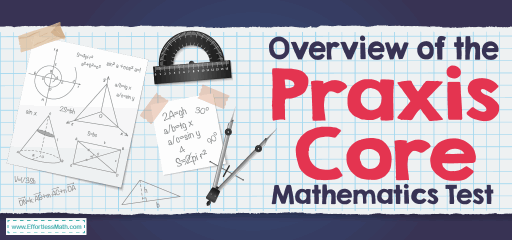# Overview of the Praxis Core Mathematics TestEveryone who wants to start teaching has to overcome some obstacles in this way, and one of the main obstacles is the Praxis Core exams. Most colleges and universities require a passing score on this set of exams to be accepted into their teacher education programs, and in most states, a passing score is required to be certified as a school teacher. The Praxis Core test, also known as the Pre-Professional Skills Test (PPST), is a test of the broad academic skills a person needs to obtain a college degree in education.

The Praxis Core test was created and operated by the Educational Testing Service and by standards put forth by the College and Career Readiness Standards, and the Common Core State Standards. Praxis Core tests are computerized tests that assess your skills in three areas: mathematics, reading, and writing. The Praxis Core tests total 152 questions and 2 essays for you in 300 minutes.

## How is the structure of the Praxis Core Mathematics test?

The math test has 56 selected-response questions and includes four areas. You will have 90 minutes to complete the math section. The math test of the Praxis Core includes:

### 1- Number and quantity (36%):

You will be tested based on your initial understanding of integers, ratios, fractions, exponents, and quantities.

### 2- Algebra and functions (20%):

You will be tested in your basic understanding of algebra and expressing your understanding of various mathematical expressions such as verbal, symbolic expressions, and diagrams. You will solve the problem by using real-life algebraic equations as well as interpreting and constructing functions.

### 3- Geometry (12%):

You will be tested for your understanding and ability to analyze geometric shapes. You will draw and explain geometric triangles, circles, surfaces, volumes, Pythagorean theorems, and modeling. Knowledge of metric measurement and US standard measurement is assumed.

### 4- Statistics and probability (32%):

You will test the ability to interpret the data and show how it matches the graphs. You then summarize, conclude, and make decisions based on the conclusions.

## Is the Praxis Core Mathematics test hard?

The Praxis Core test questions are usually much easier than the ACT and SAT questions.

## What score do you need to pass the Praxis Core Mathematics test?

The minimum scores for passing from state to state vary, the acceptance of Praxis math scores is usually 150.

## What is the highest score you can get on the math Praxis Core?

The unofficial score is also based on the Praxis Core grading scale of 100-200. It means that the lowest possible score on the Praxis Core test is 100 points, the highest possible score is 200, and the range is 100 points.

## Do you get a formula sheet on thePraxis Core Mathematics test?

Formulas are not provided in Praxis Core, so you need to know the major area, surface area, and volume formulas.

## Can you use the calculator on thePraxis Core test?

You will be provided with an on-screen, four-function calculator on the Praxis Core. The four-function calculator is a calculator that can perform basic operations like subtraction, addition, division, and multiplication. This calculator can also have functions like the square root and the exponent.

## The Best Books to Ace the Praxis Core Test

### What people say about "Overview of the Praxis Core Mathematics Test - Effortless Math: We Help Students Learn to LOVE Mathematics"?

No one replied yet.

X
52% OFF

Limited time only!

Save Over 52%

SAVE \$40

It was \$76.99 now it is \$36.99